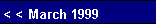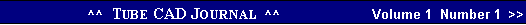Design Idea
 frequency until it reaches the DC resistance of the wire. The gain would top out at whatever would have been the gain of the tube working into the same plate resistor and with a cathode resistor equal to the DCR of the inductor. At frequencies higher than this transition frequency, the gain would keep falling, as the inductor's impedance will climb with higher frequencies and it would come to resemble a constant current source that continually traced the input voltage, which would nullify that signal, as the grid and the cathode would never experience a voltage differential. The problem with this approach is that it works too well. If the transition frequency was set 100 Hz, the signal would be -48 dB at 25 kHz, which is the same as saying that the output would be 250 times smaller at 25 kHz as it was at 100 Hz. In other words, the signal keeps attenuating and never flattens, which was our actual goal.    To achieve a shelving of the higher frequencies requires a
 The idea presented here has not been tested. It is something like a thought experiment. If you do build something along these lines, please, send us your results.Variable Feedback   Our aim here is to preserve the dynamics and openness of a feedback free amplifier at the mid and high frequencies and obtain the slam and punch of a feedback amplifier at the low end of the frequency response. Feedback has many detractors, those who argue that it steals life from the reproduction of music, leaving dull or canned sounding. But even its most vociferous opponents will admit that feedback makes for a much higher damping factor and a much tighter, well defined bass response.     In other words, having one's cake and eating it is the real goal of this design idea.     How? Frequency selective gain is the answer. By boasting the gain at the extremely low frequencies, we effectively boast the amount of feedback at those low frequencies, while still retaining a flat frequency
 that a resistor be placed in parallel with the inductor in the previous example. With the addition of this resistor the gain would be highest at low frequencies where the gain would equal that of the circuit where the cathode resistor equaled the value of the DCR of the inductor and fall off at higher frequencies until the gain reached a base gain equal to the gain the circuit would realize with just the cathode resistor in place.       An alternative approach would be to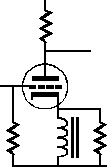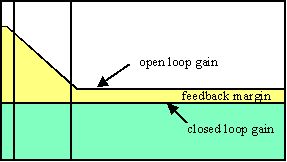+ 32 dB
 + 20 dB
 use a two-resistor voltage divider that terminates into a capacitor. The ratio between resistors would define the degree of shelving that the insertion loss of the circuit would entail.     To get the sense of this circuit, imagine both extremes: DC and infinitely high frequencies. At DC the capacitor's reactance would be infinitely high, which means the circuit would function as if the capacitor were not there at all. At
 25 kHz
 25 Hz      100 Hz
 response. Beyond the low frequencies, the obtainable feedback could be as low as 1 dB or even less. Remember that feedback compares the output with the input and adjusts the output to match the input, which means that the feedback will flatten the errant frequency response of an amplifier as well as lower its distortion.    How can the gain be increased at the low frequencies? Or
 R1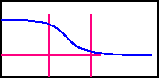another way of putting it would be How can the gain be lowered at the mid and high frequencies? One answer would be to introduce some inductance in series with the cathode of a Grounded Cathode amplifier. At the point below where the inductance's impedance  equals the rp divided by the mu of the tube sets the point below which the gain will climb, as the inductor's impedance will fall with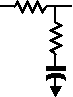0 dB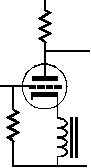R2
 loss
 C
 F1
 F2
 Loss = R2 / (R1 + R2)    F1 = 1 / 6.28·(R1 + R2)·C    F2 = 1 / 6.28·R2·Cpg. 6